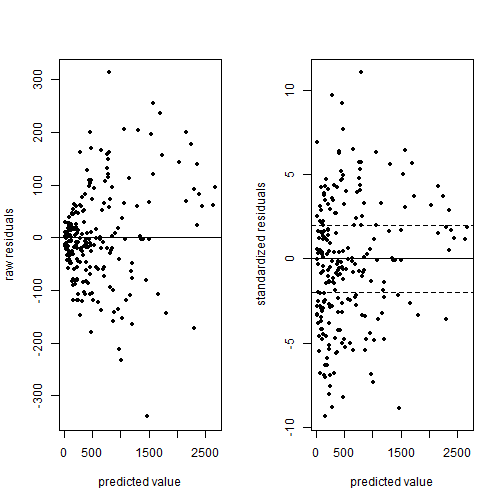# Poisson regression – Ch 6 of Gelman and Hill

Chapter 6 of Gelman and Hill's Data Analysis Using Regression and Multilevel/Hierarchical Models presents an interesting example of Poisson regression using data on police stops in New York. Put simply, they seek to model the count of “stop and frisks” as a function of ethnicity and precinct with number of arrests in the previous year included as an offset. While the example is fun and informative, it's not terribly easy to replicate. The R code is provided to run the regressions but the data is a little hard to locate. I was eventually able to find it and get everything to mostly work, so I thought I would blog about it in case anyone else was trying to do the same.

About the data: it turns out you can find it here in the “police” folder. In the folder is a dat file called “frisk_with_noise”. Here's one way to read it in:

url <- "http://www.stat.columbia.edu/~gelman/arm/examples/police/frisk_with_noise.dat"

##   stops  pop past.arrests precinct eth crime
## 1    75 1720          191        1   1     1
## 2    36 1720           57        1   1     2
## 3    74 1720          599        1   1     3
## 4    17 1720          133        1   1     4

dim(dat)

##  900   6


But wait. You'll notice the data set has 900 rows but the regression output in the book references n = 225. To replicate the example in the book, I'm pretty sure we need to aggregate over precinct and eth, like so:

stops <- aggregate(cbind(stops, past.arrests) ~ eth + precinct, data=dat, sum)


Using cbind() on stops and past.arrests allows us to sum both stops and past.arrests over all combinations of eth and precinct. Now we're ready to run the code provided in the book, which can also be found here. Here's one of the models they fit:

library(arm) # for the display() function
fit.2 <- glm (stops ~ factor(eth), data=stops, family=poisson, offset=log(past.arrests))
display(fit.2)

## glm(formula = stops ~ factor(eth), family = poisson, data = stops,
##     offset = log(past.arrests))
##              coef.est coef.se
## (Intercept)  -0.59     0.00
## factor(eth)2  0.07     0.01
## factor(eth)3 -0.16     0.01
## ---
##   n = 225, k = 3
##   residual deviance = 45437.4, null deviance = 46120.3 (difference = 682.9)


That works, but our output doesn't match the book's output! What's going on? Actually the explanation is in the title of the data file: “frisk_with_noise”. Also notice how I skipped the first 6 lines of the file when reading it in to R. Here's the first line of what I skipped:

stop and frisk data (with noise added to protect confidentiality)


So noise was apparently added after publication of the book to protect confidentiality. I guess we're not supposed to see which precincts are making the most stops of certain ethnicities? Oh well, at least we can run the code now.

We can see fitted values using the fitted() function. Here are the first 10 fitted values compared to their observed values:

cbind(observed=stops$stops[1:10], fitted=fitted(fit.2)[1:10])  ## observed fitted ## 1 202 544.2816 ## 2 102 175.7562 ## 3 81 180.0316 ## 4 132 418.2082 ## 5 144 331.8515 ## 6 71 203.6578 ## 7 752 1215.1920 ## 8 441 373.5563 ## 9 410 584.9845 ## 10 385 261.5884  Doesn't look too good to the eye, at least not the first 10. How are those fitted values calculated? Like this: $y = exp(\alpha + \beta x + log(t))$ where t is the offset, in this case, past arrrests. So to calculate the fitted value for precinct 1, ethnicity = 1 (black), and 980 arrests in the previous year, we do the following: exp(coef(fit.2) + log(980))  ## (Intercept) ## 544.2816  # or equivalently 980*exp(coef(fit.2))  ## (Intercept) ## 544.2816  # compare to R's fitted value fitted(fit.2) == exp(coef(fit.2) + log(980))  ## 1 ## TRUE  Plotting standardized residuals versus fitted values can reveal overdispersion. That's what Gelman and Hill do in figure 6.1. Below I reproduce it. First I fit a new model that includes precinct as a predictor. The figure on the left plots raw residuals versus fitted values. This is of limited use since we expect variance to increase with larger fitted values in a Poisson distribution. In other words we wouldn't expect to see a constant scatter about 0 as we would in OLS regression. The figure on the right, however, uses standardized residuals which have a mean of 0 and standard deviation of 1. If the Poisson model is true, we would expect to see most residuals falling within 2 standard deviations from 0. Many residuals falling beyond this range reveal overdispersion. And indeed that's what we see here. fit.3 <- glm (stops ~ factor(eth) + factor(precinct), data=stops, family=poisson, offset=log(past.arrests)) par(mfrow=c(1,2)) pv <- fitted(fit.3) r <- (stops$stops - fitted(fit.3))
plot(pv, r, pch=20, ylab="raw residuals", xlab="predicted value")
abline(h=0)
sr <- (stops\$stops - fitted(fit.3))/sqrt(fitted(fit.3))
plot(pv, sr, pch=20, ylab="standardized residuals", xlab="predicted value")
abline(h=c(-2,0,2),lty=c(2,1,2))par(mfrow=c(1,1))


To correct for overdisperson in R you can repeat the code for fit.3 above with family = quasipoisson. This corrects the standard errors of the regression coefficients by making them larger.